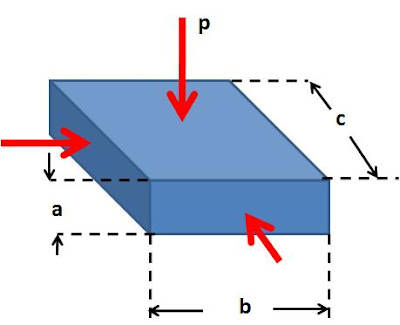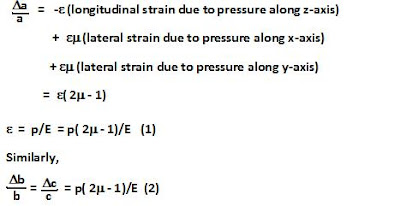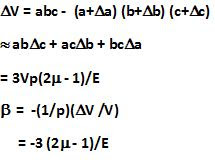## Wednesday, April 8, 2009

### Irodov Problem 1.299Liquids exert equal pressure in all directions, thus the body experiences equal pressure p from all directions. Suppose that the dimensions of the body are a,b, and c as shown in the figure.

Each side experiences a compression due to the pressure along its direction (dictated by Young's modulus) and expansion due to lateral strain (dictated by the Poisson's ratio).

Hence, we have,The change in volume is given by,From he above expression it seems as though for a value of Poisson's ratio that is greater than 0.5, the volume of the body will actually increase. Since the body is being compressed from all directions, its volume cannot possibly increase. Thus, we conclude that the value of Poisson's ratio cannot be greater than 0.5.